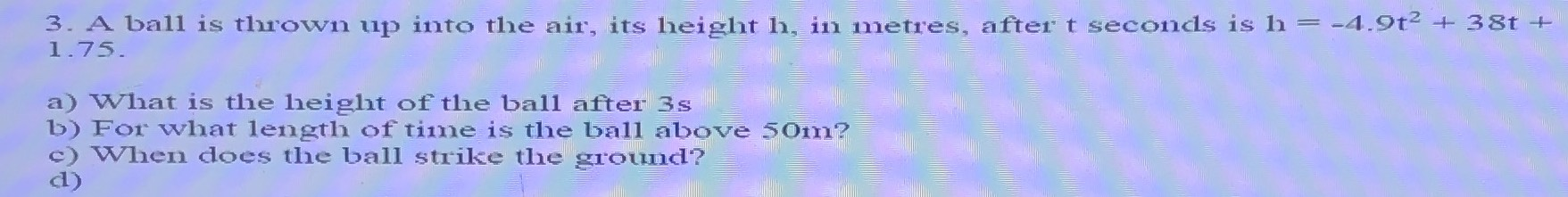### ¿Todavía tienes preguntas de matemáticas?

Pregunte a nuestros tutores expertos
Algebra
Pregunta3. A ball is thrown up into the air, its height h, in metres, after t seconds is $$h = - 4.9 t ^ { 2 } + 38 t +$$ $$1.75$$ .

a) What is the height of the ball after $$3 s$$

b) For what length of time is the ball above $$50 m$$ ?

c) When does the ball strike the ground?